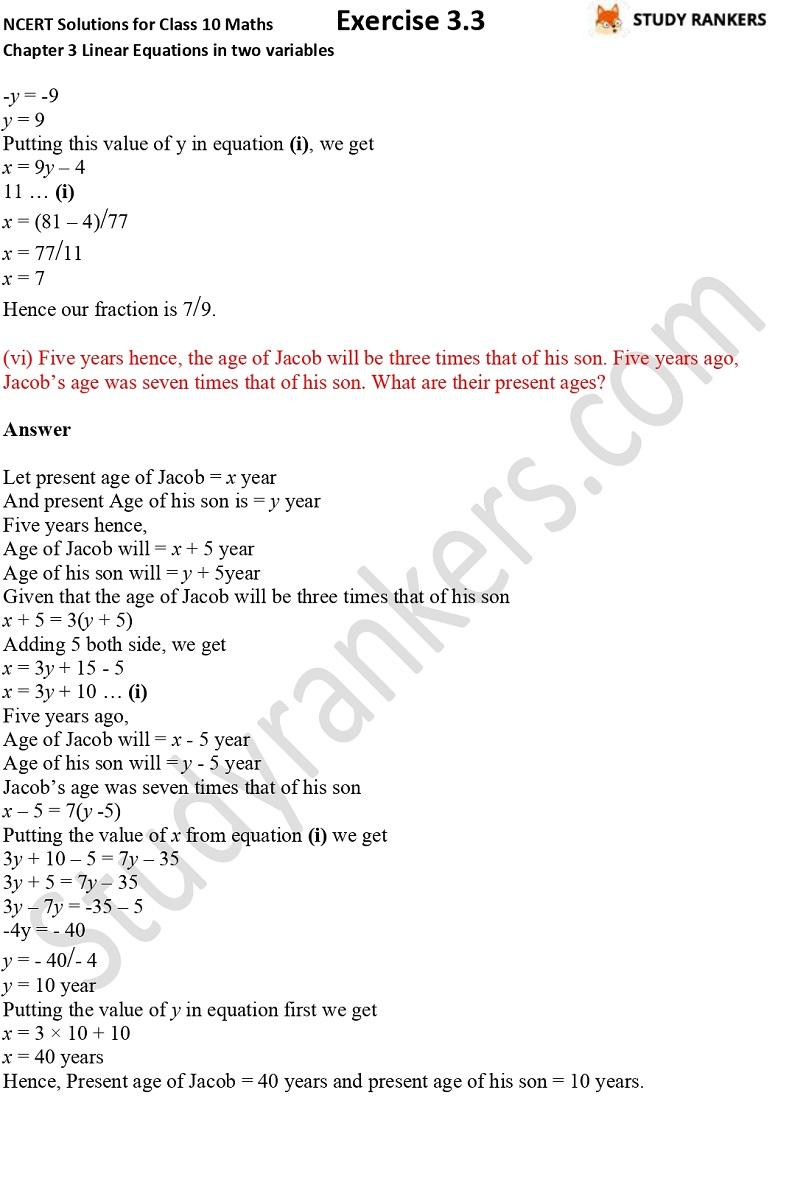## NCERT Solutions for Class 10 Maths Chapter 3 Pair of Linear Equations in Two Variables Exercise 3.3

Here we are giving Chapter 3 Pair of Linear Equations Class 10 Maths NCERT Solutions that will help you in passing the exams with flying colors. Chapter 3 NCERT Solutions Class 10 Maths can be used for solving your homework and understanding the concepts and formula in the chapter. The solutions are updated as per the latest marking scheme provided by CBSE 2019-20.